# Control of Flow

## Overview

VBScript code executes in a top-down manner. The runtime engine starts at the top of a script and processes one line at time until it reaches the end of the script. However, this does not mean that every single line of code in that script will execute, and furthermore, some lines of code will execute more than once-thousands of times even. Some lines are skipped because of branching logic that tells the runtime engine to skip a certain block of code. Other lines are executed multiple times because of looping logic that repeats the same block of code over and over.

Branching logic is implemented in VBScript with statements such as If , Else , and Select Case . Loops are defined with the For , Do , and While statements. The sections in this chapter will prepare you with all of the information you need on branching and looping, which are as essential to programming as variables . If you are relatively new to programming, this will be an important chapter. Like all of the chapters up to this point, this chapter explains essential programming fundamentals while also teaching you the VBScript-specific techniques and syntax.

If you are an experienced programmer in another language, you might only skim this chapter for some of the VBScript particulars. VBScript's branching and looping capabilities are basically the same as any mature procedural language. If you are looking only for syntax details, the language reference in Appendix A might be your best source of information.

## Branching Constructs

Branching is the process of making a decision in your code and then, based on that decision, executing one block of code, but not others. If you have been reading along since the beginning of the book, you have seen the most common branching construct, If End If , many times already. In this chapter, we will cover in detail If End If along with another branching construct, Select End Select .

If End If and Select End Select are both used to define a code block , which is a section of code that is bounded by beginning and ending statements. In the case of an If block, the beginning of it is defined by an If statement, and the end is defined by an End If statement. Select End Select follows the same pattern. VBScript requires that both the beginning and the ending statements be there. If you forget to include the ending statement, VBScript will produce a syntax error at runtime.

It's a good idea to get in the habit of typing both the beginning and ending statements first, before you type the code that goes between them. This ensures that you will not forget to type the ending statement, especially if the code that goes between the statements is rather involved. This is also especially helpful if you're going to be nesting multiple code blocks within each other.

### The If Branch

The If End If construct can be very simple, or it can become fairly complicated. In its simplest form, it requires this syntax.

```

If  Then

End If

```

In place of < expression > you can use anything that results in a True or False answer (also known as a Boolean expression). This can be a mathematical equation.

```

If 2 + 2 = 4 Then

End If

```

Or it can be a function that returns True or False .

```

If IsNumeric(varAny) Then

End If

```

Or it can use more complicated Boolean logic.

```

If strMagicWord = "Please" And _

(strName = "Hank" Or strName = "Bill") Then

End If

```

You can also use the Not statement to reverse the True or False result of the expression.

```

If Not IsNumeric(varAny) Then

End If

```

We can add another dimension to the If construct by adding an Else block. The Else block will be executed if the result of the If expression is False .

```

If IsNumeric(varAny) Then

Else

End If

```

Many times, however, the decision you are trying to make does not involve a simple either/or evaluation. In that case, you can add as many ElseIf blocks as you like.

```

If IsNumeric(varAny) Then

ElseIf IsDate(varAny) Then

ElseIf IsEmpty(varAny) Then

Else

End If

```

If the first expression returns False , then the execution moves to the first ElseIf evaluation. If that returns False , then the execution moves on to the second ElseIf evaluation. If that returns False , then the execution falls into the code in the Else block. The ElseIf line must end with the word Then , just as the initial If line must. The Else block is always optional and must come last.

```

If IsNumeric(varAny) Then

ElseIf IsDate(varAny) Then

ElseIf IsEmpty(varAny) Then

End If

```

You can also nest If End If blocks within each other.

```

If IsNumeric(varAny) Then

If varAny > 0 Then

```
``````
``````

ElseIf varAny < 0 Then

Else

End If

Else

End If

``````

`You can nest as deeply as you like, but beware of nesting too deeply, because the logic of the code can become unmanageable and hard to follow. `

`Keep in mind that a Select End Select block (which we will introduce in the next section) is often an alternative to an If End If block with a lot of ElseIf clauses in the middle. However, the ElseIf construct is more flexible, because each different ElseIf line can evaluate something totally different, whereas a Select End Select block must consider different possible results to the same expression. Because the If ElseIf End If is more flexible, you can always use it in place of Select End Select . However, the reverse is not true. Select End Select can only be used to evaluate different variations of the same expression. `

`Here is a sequence of ElseIf blocks that evaluate totally different expressions. `

``````

If boolFirst Then

ElseIf boolSecond Then

ElseIf boolThird Then

ElseIf lngTest = 1 Then

ElseIf strName = "Bill" Then

End If

``````

### `The Select Case Branch`

`As we mentioned in the previous section, the Select End Select construct is useful when you are evaluating different possible results to the same expression. Select End Select has the following syntax.`

``````

Select Case

Case

Case

Case

Case

Case Else

End Select

``````

`Notice that we are evaluating the same expression multiple times, whereas the If ElseIf End If block allows you to evaluate different expressions. Notice also that after all of the tests are made, we can include an optional Case Else block that will be executed if none of the other possibilities return True . Let's look at a more concrete example. `

``````

Select Case VarType(varAny)

Case vbString

Case vbLong

Case vbBoolean

Case Else

End Select

``````

`The first line evaluates the expression VarType(varAny); then each subsequent Case statement checks for each of many possible results. Finally, if none of the Case statements evaluates to True , then the Case Else block will be executed. Note that we could accomplish this same thing with an If ElseIf End If block. `

``````

If VarType(varAny) = vbString Then

ElseIf VarType(varAny) = vbLong Then

ElseIf VarType(varAny) = vbBoolean Then

Else

End If

``````

`However, this has the disadvantage that the expression VarType(varAny) will be executed for every ElseIf block, whereas with the Select End Select , it is evaluated only once, which is more efficient. Some programmers would also argue that the Select Case block is more elegant and readable than a series of ElseIf statements. `

`It is a good idea to always consider including a Case Else block in your Select Case blocks-even if you cannot conceive of a situation where the Case Else would be executed. This is a good idea for two reasons. `

`First, if the input data or code for your script changes unexpectedly, and the Case Else block does suddenly start executing, your code will catch it-whereas without the Case Else block you might never catch it. This is useful when you are expecting a limited set of input values that you are checking for, with the Case Else block catching any other input data that does not match the expected set of values. `

`Second, including a Case Else block can add documentation to the code as to why the Case Else block is never intended to be executed. It's a common convention to include a Case Else block that contains nothing other than a comment stipulating why the programmer expects the Else condition to never exist. Here's an example that uses both a comment and an error message. `

``````

Select Case lngColor

Case vbRed

Case vbGreen

Case vbBlue

Case Else

'We never use anything but Red, Green, and Blue

MsgBox "Illegal color encountered: " & lngColor, _

vbExclamation

End Select

``````

`You can also nest Select End Select blocks within one another, and you can nest If End If blocks (or any other kind of code) inside the Select End Select as well. `

``````

Select Case VarType(varAny)

Case vbString

Select Case varAny

Case "Test1"

Else

End If

Case "Test2"

Case "Test3"

End Select

Case vbLong

Case vbBoolean

Case Else

End Select

``````

` `

## `Loop Constructs`

`Branching is the process of making a decision on whether to execute one block of code or another. Looping is the process of repeating the same block of code over and over. `

`VBScript provides four looping constructs that you can use in different situations. In the view of most Visual Basic and VBScript programmers, however, one of these loop constructs, the While Wend loop, has been supplanted by the more intuitive, powerful, and flexible Do Loop loop. For this reason, in this chapter we will emphasize the remaining three loops . However, in the interest of completeness, we will cover the syntax for the While Wend loop at the end of the chapter. `

`Once you remove While Wend from consideration, each of the remaining three loop constructs is ideal for a different type of loop. Each of the following sections will explain the syntax for these loops, as well as when you would use one loop or another. `

### `For Next`

`The For Next loop is ideal for two situations: first, when you want to execute a block of code repeatedly a known, finite number of times; and second, when you want to execute a block of code once for each element in a complex data structure such as an array, file, or database table. (However, the For Each Next loop is specifically designed for another kind of complex data structure, the collection .) `

`Let's first look at how to use the For Next loop to execute a block of code a known number of times ( FOR_LOOP_SIMPLE.VBS ). `

``````

Option Explicit

Dim lngIndex

For lngIndex = 1 To 5

MsgBox "Loop Index: " & lngIndex

Next

``````

`Running this code produces, in succession, the five dialog boxes shown in Figure 5-1 through 5-5. `

```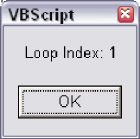Figure 5-1 ```

```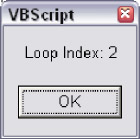Figure 5-2 ```

```Figure 5-3 ```

```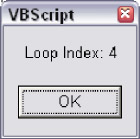Figure 5-4 ```

```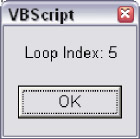Figure 5-5 ```

`This is pretty straightforward. The first thing you'll notice is that in order to use the For Next loop, you need a loop variable-also known as a loop index. The variable lngIndex serves this purpose. The statement For lngIndex = 1 To 5 means that this loop will execute five times. As you can see from the dialog boxes that appear, the value of lngIndex matches each step in the traversal from the number 1 to the number 5 . After looping for the fifth time, the loop stops and the code moves on. Note that you don't need to start at 1 in order to loop five times ( FOR_LOOP_NOT_ONE.VBS ).`

``````

Option Explicit

Dim lngIndex

For lngIndex = 10 To 14

MsgBox "Loop Index: " & lngIndex

Next

``````

`This will still loop five times, but instead of starting at 1 , it will start at 10 . As the loop iterates, lngIndex will have a value of 10 , then 11 , then 12 , and so on to 14 .`

`You can also use the Step keyword to skip numbers ( FOR_LOOP_STEP.VBS ).`

``````

Option Explicit

Dim lngIndex

For lngIndex = 10 To 18 Step 2

MsgBox "Loop Index: " & lngIndex

Next

``````

`Once again, this will still loop five times, but, because we specified Step 2 , it will skip every other number. On the first loop, lngIndex will have a value of 10 , then 12 , then 14 , and so on to 18 . You can use any increment you like with the Step keyword ( FOR_LOOP_STEP_100.VBS ).`

``````

Option Explicit

Dim lngIndex

For lngIndex = 100 To 500 Step 100

MsgBox "Loop Index: " & lngIndex

Next

``````

`You can also use the Step keyword to cause the loop to go backwards ( FOR_LOOP_BACKWARDS .VBS ).`

``````

Option Explicit

Dim lngIndex

For lngIndex = 5 To 1 Step -1

MsgBox "Loop Index: " & lngIndex

Next

``````

`Because we used a negative number with the Step keyword, the loop goes downward through the numbers. Notice that in order for this to work, the increment range must specify the larger number first.`

`You are not limited to using negative numbers with the Step keyword. The loop itself can loop through negative numbers, like this ( FOR_LOOP_NEGATIVE.VBS ):`

``````

Option Explicit

Dim lngIndex

For lngIndex = -10 To -1

MsgBox "Loop Index: " & lngIndex

Next

``````

`Or like this ( FOR_LOOP_NEGATIVE2.VBS ):`

``````

Option Explicit

Dim lngIndex

For lngIndex = -10 To -20 Step -2

MsgBox "Loop Index: " & lngIndex

Next

``````

`You can also nest loops inside one another ( FOR_LOOP_NESTED.VBS ).`

``````

Option Explicit

Dim lngOuter

Dim lngInner

For lngOuter = 1 to 5

MsgBox "Outer loop index: " & lngOuter

For lngInner = 10 to 18 Step 2

MsgBox "Inner loop index: " & lngInner

Next

Next

``````

`So what do you do when you don't know exactly how many times you want to loop? This is a common situation. It often comes up when you need to traverse an array (see Chapter 3), a string, or any other kind of structure. Let's look at an example ( EXTRACT_FILE_NAME.VBS ).`

``````

Option Explicit

Dim lngIndex

Dim lngStrLen

Dim strFullPath

Dim strFileName

'This code will extract the filename from a path

strFullPath = "C:WindowsTempTestmyfile.txt"

lngStrLen = Len(strFullPath)

For lngIndex = lngStrLen To 1 Step -1

If Mid(strFullPath, lngIndex, 1) = "" Then

strFileName = Right(strFullPath, _

lngStrLen - lngIndex)

Exit For

End If

Next

MsgBox "The filename is: " & strFileName

``````

`Running this code produces the dialog box shown in Figure 5-6.`

```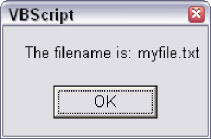Figure 5-6 ```

`We've added some new elements in this example. The Len() function is a built-in VBScript function that returns the number of characters in a string. The Mid() function extracts one or more bytes from the middle of a string. The first parameter is the string to extract from; the second parameter is the character at which to start the extraction; the third parameter is how many characters to extract. The Right() function is similar to Mid() , except that it extracts a certain number of the rightmost characters in a string. Finally, the Exit For statement breaks you out of a loop. This is very handy when you know that you don't need to loop anymore.`

`Notice how we use the length of the strFullPath variable to drive how many times we need to loop. When we started, we did not know how many times we needed to loop, so we used the length of the structure we needed to traverse (in the case, a string) to tell us how many times to loop. Notice also how we loop backwards so that we can search for the last backslash character ('') in the strFullPath variable. Once we've found the backslash, we know where the filename begins. Once we've used the Right() function to extract the filename into the strFileName variable, we don't need the loop anymore (we've accomplished our goal), so we use Exit For to break out of the loop. Exit For jumps the execution of the code to the very next line after the Next statement.`

 `Note  ` `The above example was provided for the purpose of demonstrating how to use a For Next loop to move through a data structure of a size that is unknown at design time. This is not necessarily the best way to extract a filename from a full pathname. This, for example, would be much faster (EXTRACT _ FILE _ NAME _ NO _ LOOP.VBS) .` `````` Option Explicit Dim strFileName Dim strFullPath strFullPath = "C:MyStuffDocumentsPersonal esume.doc" strFileName = Right(strFullPath, _ Len(strFullPath) - InStrRev(strFullPath,"")) MsgBox "The filename is: " & strFileName ``````

`There is almost always more than one way to solve the same problem. Loops are very handy and an integral part of programming, but they are also expensive from a performance standpoint. The second example is better for two reasons: one, there are less lines of code; and two, since it does not use a loop to repeat the same lines of code over and over, it find the answer much faster.`

### `For Each Next`

`The For Each Next loop is a special kind of loop that is specifically used for traversing collections. A 'collection,' as the name suggests, is a collection of data, almost like an array. A 'collection' is most often a collection of objects of the same type (even though 'collections' can be collections of virtually any kind of data).`

`For example, built into the VBScript 'runtime objects' FileSystemObject (see Chapter 7) is the Folder object, which represents a directory in a file system. The Folder object has a Files collection, which is exposed as a property on the Folder object. Inside the Folder.Files collection are zero or more File objects. You can use a For Each Next loop to move through each of the File objects in the Folder.Files collection.`

`With the For Each Next loop, you cannot directly control how many times the loop will go around. This is dependent upon how many objects are in the collection you are traversing. However, you can still use the Exit For statement to break out of the loop at any time. You can figure out when to use Exit For by testing for some condition, or using an extra counter variable to count how many times you've gone through the loop.`

`Let's look at an example that uses the FileSystemObject and related objects, which we introduce formally in Chapter 7. In this example ( FSO_FIND_FILE.VBS ), we will attempt to locate the AUTOEXEC.BAT file on our system. (Don't worry-it's safe to try out this code-there is no danger of harming your AUTOEXEC.BAT file.)`

``````

Option Explicit

Dim objFSO

Dim objRootFolder

Dim objFileLoop

Dim boolFoundIt

Set objFSO = _

WScript.CreateObject("Scripting.FileSystemObject")

Set objRootFolder = objFSO.GetFolder("C:")

Set objFSO = Nothing

boolFoundIt = False

For Each objFileLoop In objRootFolder.Files

If UCase(objFileLoop.Name) = "AUTOEXEC.BAT" Then

boolFoundIt = True

Exit For

End If

Next

Set objFileLoop = Nothing

Set objRootFolder = Nothing

If boolFoundIt Then

MsgBox "We found your AUTOEXEC.BAT file in "& _

"the C: directory."

Else

MsgBox "We could not find AUTOEXEC.BAT in " & _

"the C: directory."

End If

``````

`Don't worry about any syntax that may be unfamiliar to you. Concentrate instead on the syntax of the For Each Next loop block. The objRootFolder variable holds a reference to a Folder object. A Folder object has a Files collection. The Files collection is a collection of File objects. So what VBScript is telling us to do is 'take a look at each File object in the Files collection.' Each time the loop goes around, the loop variable, objFileLoop , will hold a reference to a different File object in the Files collection. If the Files collection is empty, then the loop will not go around at all. Notice how we use the Exit For statement to break out of the loop once we've found the file we're looking for.`

`The last script example is intended to demonstrate the use of the For Each Next loop to traverse a collection of objects. Just as in the previous section, using a loop in this way is not necessarily the best way to see if a file exists. For example, this is much faster and more compact ( FSO_FIND_FILE_NO_LOOP.VBS ).`

``````

Option Explicit

Dim objFSO

Set objFSO = _

WScript.CreateObject("Scripting.FileSystemObject")

If objFSO.FileExists("C:AUTOEXEC.BAT") Then

MsgBox "We found your AUTOEXEC.BAT file in the " & _

"C: directory."

Else

MsgBox "We could not find AUTOEXEC.BAT in " & _

"the C: directory."

End If

Set objFSO = Nothing

``````

`You might be getting the idea that we're trying to send the message that you should not use loops, that there is always a better way. This is not the case. Loops are extremely useful and many well-written scripts will use them often. Programming is most often about using some kind of data, and often meaningful data is stored in complex structures like arrays and collections. If you need to root around inside that data to do what you need to do, the loop is your friend. However, as we mentioned, many times a loop seems like the obvious solution, but there may be a more elegant, less expensive alternate solution.`

`Before we move on to the Do loop, please note that even though the For Each Next loop is most often used to loop through collections, it can also be used to loop through all of the elements of an array. No matter how many elements or dimensions the array has, the For Each Next loop will touch each and every one of them. Here is an example of using the For Each Next loop to traverse a single dimension array ( FOR_EACH_ARRAY.VBS ).`

``````

Option Explicit

Dim astrColors(3)

Dim strElement

astrColors(0) = "Red"

astrColors(1) = "Green"

astrColors(2) = "Blue"

astrColors(3) = "Yellow"

For Each strElement In astrColors

MsgBox strElement

Next

``````

### `Do Loop`

`Do loop is the most versatile of all of the loop constructs. This is because you can easily make it loop as many times as you like based on any criteria you like. (However, you'd have to go through some trouble to use it to traverse a collection- For Each Next is much better for that.) The power of the Do loop is in the use of the While and Until keywords. You can use While or Until at either the beginning of the loop or the end of the loop to control whether the loop will go around again. Let's look at a simple script that uses a Do loop ( DO_WHILE.VBS ).`

``````

Option Explicit

Dim boolLoopAgain

Dim lngLoopCount

Dim strResponse

boolLoopAgain = False

lngLoopCount = 0

Do

boolLoopAgain = False

lngLoopCount = lngLoopCount + 1

strResponse = InputBox("What is the magic word?")

MsgBox "Correct! Congratulations!"

Else

If lngLoopCount < 5 Then

MsgBox "Sorry, try again."

boolLoopAgain = True

Else

MsgBox "Okay, the word we wanted was 'Please'."

End If

End If

Loop While boolLoopAgain

``````

`Using a Do loop in this way to process user input is a common technique. You need to ask the user something, but he or she might enter illegal data. You need a way to check the input and, if necessary, loop back and ask the user to enter it again.`

`Notice how the Do statement marks the beginning of the loop block, and how the Loop statement defines the end of the block. The While statement, however, places a condition on the Loop statement. The loop will only go around again if the expression following the While statement is True . In this case, our expression is a variable called boolLoopAgain , which has the Boolean subtype, but it could be any expression that evaluates to or returns a True or False response.`

`Notice also how we initialize the boolLoopAgain variable to False before the loop starts. This accomplishes two things: it establishes the subtype of the variable as Boolean , and it guarantees that the loop will only go around again if some piece of code inside the loop explicitly sets the variable to True . If the user guesses wrong, then we set boolLoopAgain to True , guaranteeing that the loop will go around at least one more time and so we can ask the user to guess again.`

`Finally, notice how we use a loop counter variable, lngLoopCount , to make sure that the loop does not go around forever and drive the user crazy if he or she can't guess the magic word. Using a loop counter variable is optional, and not part of the Do Loop syntax, but it's a good idea if there's a chance that the loop might go around indefinitely.`

`Using this particular loop structure-with the Do statement by itself at the beginning, and the While condition attached to the Loop statement at the end-has an important implication : because we did not place a condition on the Do statement, the code inside the loop is guaranteed to execute at least once . This is what we want in this case, because if we did not execute the code at least one time, the user would never get asked the question "What is the magic word?" `

`Sometimes, though, you only want the code inside the loop to execute if some precondition is True; if that precondition is False , then you don't want the loop to execute at all. In that case, we can place the While statement at the beginning of the loop. If the Do While condition is False , then the loop will not go around even once.`

`In the following example, we are going to use the FileSystemObject to open a text file. We will access the text file using a TextStream object. When you open a file in the form of a TextStream object, the TextStream object uses a 'pointer' to keep track of it's place in the file as you move through it. When you first open the file, the pointer is at the beginning of the file. (The pointer is not physically placed in the file-it exists only in memory in the TextStream object.) You can move through the file line by line using the TextStream.ReadLine method.`

`Each time you call ReadLine , the pointer moves one line down in the file. When the pointer moves past the last line in the file, the TextStream.AtEndOfStream property will have a value of True . That's when we know we are done reading the file. There is a possible problem, though: when we open a text file, we're not sure if it actually contains any data. It might be empty. If it is, then we don't want to call ReadLine , because this will cause an error. However, we'll know that the file is empty if the AtEndOfStream property is True right after opening the file. We can handle this nicely by placing the calls to ReadLine inside of a Do loop.`

`If you want to try out this code yourself, just create a text file and put the following lines in it (the downloadable code contains a file called TESTFILE.TXT ).`

``````Line 1
Line 2
Line 3
Line 4
Line 5
``````

`Save the file to your hard drive in the same location as the script below ( DO_WHILE_READ_FILE.VBS ). The script assumes that TESTFILE.TXT is in the same directory as the script file. While you're reading this code, don't worry if you're not familiar with the particulars of the FileSystemObject and TextStream objects, which are covered in detail in Chapter 7. Just pay attention to the way we use the While condition in conjunction with the Do statement.`

``````

Option Explicit

Dim objFSO

Dim objStream

Dim strText

Set objFSO = _

WScript.CreateObject("Scripting.FileSystemObject")

Set objStream = objFSO.OpenTextFile("testfile.txt")

Set objFSO = Nothing

strText = ""

Do While Not objStream.AtEndOfStream

strText = strText & objStream.ReadLine & vbNewLine

Loop

Set objStream = Nothing

If strText <> "" Then

MsgBox strText

Else

MsgBox "The file is empty."

End If

``````

`Running this code results in the dialog box shown in Figure 5-7.`

```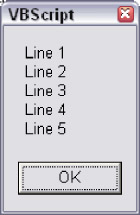Figure 5-7 ```

`You can see that by placing the While condition at the beginning of our loop, we can decide whether or not we want the loop to go around even once. If the file is empty, then we don't want to try reading any lines. Since there is no condition on the Loop statement, though, when the loop reaches the end, the code will jump back up to the Do line. However, if the Do While expression returns False , the loop will not execute again, and the code will jump back down to the line immediately following the Loop line.`

`The objStream.AtEndOfStream property will be True only when the end of the file is reached. As long as we have not reached the end of the file, we want to keep looping. If we start out at the end of the file because the file is empty, we don't want to loop at all.`

`Going back to our first Do loop example, for the record, note that we could have put the While statement with the Do in our first example and accomplished the same thing ( DO_WHILE2.VBS ).`

``````

Option Explicit

Dim boolLoopAgain

Dim lngLoopCount

Dim strResponse

boolLoopAgain = True

lngLoopCount = 0

Do While boolLoopAgain

boolLoopAgain = False

lngLoopCount = lngLoopCount + 1

strResponse = InputBox("What is the magic word?")

MsgBox "Correct! Congratulations!"

Else

If lngLoopCount < 5 Then

MsgBox "Sorry, try again."

boolLoopAgain = True

Else

MsgBox "Okay, the word we wanted was 'Please'."

End If

End If

Loop

``````

`Compare our first Do loop example with this one. Both examples accomplish exactly the same thing: the loop executes at least once, and it will only loop again if the code inside the loop says that we should. The difference with this second technique is that we started off by initializing boolLoopAgain to True , which guarantees that the loop will execute at least once.`

`As you can see, the Do loop is quite versatile, and how you accomplish one thing or another is largely a matter of preference. That said, one could make a pretty good argument that the first version of this code is preferable because the Do statement all by itself makes it obvious that the loop is going to execute at least once, whereas this second example is a little bit tricky.`

 `Note  ` `All else being equal, if there are two ways of coding something, the more explicit method is almost always preferable.`

`So the first question you need to answer when considering the use of the Do loop is, do I want the code to execute at least once, no matter what? If the answer to this question is yes, then it's best to place your condition at the end of the loop. Otherwise, put the condition at the beginning of the loop.`

`However, there is a second question: should you use the While statement for the condition, or its cousin, the Until statement? The answer to this second question is also largely a matter of preference. Although the While and Until statements are slightly different, they pretty much do the same thing. The main difference is one of semantics, and people generally fall into the habit of using one or the other, based on which syntax makes the most intuitive sense to them. However, one will usually tend to be more clear than another in a given situation.`

`Here's how Microsoft's VBScript documentation describes the Do loop (we added the bold emphasis).`

 `Tip  ` `Repeats a block of statements while a condition is True or until a condition becomes True.`

`As you can see, the distinction between While and Until is rather fuzzy. The easiest way to explain the difference is to modify our previous two examples replacing While with Until . You'll see that the consideration of whether to execute the loop at least once remains the same. However, the implementation is slightly different. Here's our first example, modified to use Until instead of While ( DO_UNTIL.VBS ).`

``````

Option Explicit

Dim boolLoopAgain

Dim lngLoopCount

Dim strResponse

boolLoopAgain = False

lngLoopCount = 0

Do

boolLoopAgain = False

lngLoopCount = lngLoopCount + 1

strResponse = InputBox("What is the magic word?")

MsgBox "Correct! Congratulations!"

Else

If lngLoopCount < 5 Then

MsgBox "Sorry, try again."

boolLoopAgain = True

Else

MsgBox "Okay, the word we wanted was 'Please'."

End If

End If

Loop Until boolLoopAgain = False

``````

`It may look like the same code, but the difference is that we must test for a False value in our Until clause, whereas we tested for a True value in our While clause. When you read the line Loop While boolLoopAgain , does it make more sense than Loop Until boolLoopAgain = False ? If the While syntax makes more sense to you, maybe we can fix that by changing the name of our variable ( DO_UNTIL_BETTER_NAME.VBS ).`

``````

Option Explicit

Dim boolStopLooping

Dim lngLoopCount

Dim strResponse

boolStopLooping = True

lngLoopCount = 0

Do

boolStopLooping = True

lngLoopCount = lngLoopCount + 1

strResponse = InputBox("What is the magic word?")

MsgBox "Correct! Congratulations!"

Else

If lngLoopCount < 5 Then

MsgBox "Sorry, try again."

boolStopLooping = False

Else

MsgBox "Okay, the word we wanted was 'Please'."

End If

End If

Loop Until boolStopLooping = True

``````

`Does the Until syntax make a little more sense now? The point is you can use either While or Until to accomplish what you need to-it's just a matter of what makes more sense in a given situation. Let's look at our file reading example again, this time using Until instead of While ( DO_UNTIL_READ_FILE.VBS ).`

``````

Option Explicit

Dim objFSO

Dim objStream

Dim strText

Set objFSO = _

WScript.CreateObject("Scripting.FileSystemObject")

Set objStream = objFSO.OpenTextFile("testfile.txt")

Set objFSO = Nothing

strText = ""

Do Until objStream.AtEndOfStream

strText = strText & objStream.ReadLine & vbNewLine

Loop

Set objStream = Nothing

If strText <> "" Then

MsgBox strText

Else

MsgBox "The file is empty."

End If

``````

`The Until syntax might make this more clear. People sometimes have an easier time thinking in terms of positives, and the syntax Do While Not objStream.AtEndOfStream may be more or less clear to you than Do Until objStream.AtEndOfStream . It's up to you, though. VBScript doesn't care. And if you use good variable names , stick to straightforward logic, and make good use of indenting and white space, your fellow programmers most likely won't care either.`

`Before we move on to While Wend , we need to mention the Exit Do statement. Like Exit For , you can use Exit Do to break out of a Do loop at any point. You can have as many Exit Do statements inside your loop as you like. Here's an example, yet another variation on our 'magic word' example ( DO_WHILE3.VBS ).`

``````

Option Explicit

Dim boolLoopAgain

Dim lngLoopCount

Dim strResponse

boolLoopAgain = False

lngLoopCount = 0

Do

boolLoopAgain = False

lngLoopCount = lngLoopCount + 1

strResponse = InputBox("What is the magic word?")

MsgBox "Correct! Congratulations!"

Exit Do

Else

If lngLoopCount < 5 Then

MsgBox "Sorry, try again."

boolLoopAgain = True

Else

MsgBox "Okay, the word we wanted was 'Please'."

Exit Do

End If

End If

Loop While boolLoopAgain

``````

`Instead of setting boolLoopAgain to False , we just execute an Exit Do , which has the same effect in that we won't go around the loop again. When the Exit Do statement executes, the code jumps out of the loop, to the line of code immediately following the last line of the loop block (in our example, there is not any code after our loop, so the script ends). However, while this example illustrates the proper syntax for Exit Do , we have not necessarily made our magic word code any better by using it.`

`Remember the procedures and functions discussion in Chapter 4? When discussing the Exit Sub and Exit Function statements, we said that you should use them carefully and that there is usually a way to organize your code so that you do not have to use them. The potential problem with using Exit Sub and Exit Function is that the logic can become hard to follow because of the jumping out of the flow. The same principle applies to Exit Do .`

`If you compare the original magic word code to this new version, in the original we used the boolLoopAgain statement in conjunction with If conditions to control the loop. The logic flows from top to bottom in a linear fashion. Our new code with the Exit Do statements has lost that elegance and clarity.`

`A final note about Exit Do (and the other loop Exit statements as well): if you are working with nested loops, an Exit Do executed in the inner loop does not break out of the outer loop as well-only from the loop in which the Exit Do was executed.`

### `While Wend`

`As we mentioned at the beginning of the chapter, the While Wend loop is an older loop syntax from early versions of BASIC and Visual Basic. The Do loop (see previous section) is often preferred by programmers over the While Wend loop, which is not nearly as versatile. This is not to say that it is not perfectly valid to use it, and many programmers use it often. It works fine, it's simple, and Microsoft certainly has not given any indication that they plan to remove support for it. It has simply fallen out of vogue . In the interest of completeness, here's an example of the While Wend syntax ( WHILE_WEND.VBS ).`

``````

Option Explicit

Dim lngCounter

lngCounter = 0

While lngCounter <= 20

lngCounter = lngCounter + 1

'

Wend

``````

`Unlike the Do loop, you do not have the option of using either While or Until , nor can you place the condition at the end of the loop. The condition for whether to loop again can only be placed at the beginning of the loop, as you see here. Finally, a significant limitation of the While Wend loop is that there is no equivalent to Exit For or Exit Do , meaning you cannot forcibly break out of the loop.`

` `

## `Summary`

`In this chapter we covered the topic of 'control of flow,' which involves branching and looping. Branching is the technique of checking conditions, making a decision, and executing (or not executing) a block of code based on that decision. Following are the branching constructs in VBScript.`

``````If ElseIf Else End If
Select Case End Select
``````

`Looping is the technique of repeating the same block of code over again. The looping constructs in VBScript are as follows .`

``````For Next
For Each Next
Do Loop While
Do While Loop
Do Loop Until
Do Until Loop
While Wend
``````

` `

# `Chapter 6 Error Handling and Debugging`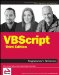VBScript Programmers Reference
ISBN: 0470168080
EAN: 2147483647
Year: 2003
Pages: 242